# Numpy core libraries¶

Starting from numpy 1.3.0, we are working on separating the pure C, “computational” code from the python dependent code. The goal is twofolds: making the code cleaner, and enabling code reuse by other extensions outside numpy (scipy, etc...).

## Numpy core math library¶

The numpy core math library (‘npymath’) is a first step in this direction. This library contains most math-related C99 functionality, which can be used on platforms where C99 is not well supported. The core math functions have the same API as the C99 ones, except for the npy_* prefix.

The available functions are defined in npy_math.h - please refer to this header in doubt.

### Floating point classification¶

NPY_NAN
This macro is defined to a NaN (Not a Number), and is guaranteed to have the signbit unset (‘positive’ NaN). The corresponding single and extension precision macro are available with the suffix F and L.
NPY_INFINITY
This macro is defined to a positive inf. The corresponding single and extension precision macro are available with the suffix F and L.
NPY_PZERO
This macro is defined to positive zero. The corresponding single and extension precision macro are available with the suffix F and L.
NPY_NZERO
This macro is defined to negative zero (that is with the sign bit set). The corresponding single and extension precision macro are available with the suffix F and L.
int npy_isnan(x)
This is a macro, and is equivalent to C99 isnan: works for single, double and extended precision, and return a non 0 value is x is a NaN.
int npy_isfinite(x)
This is a macro, and is equivalent to C99 isfinite: works for single, double and extended precision, and return a non 0 value is x is neither a NaN or a infinity.
int npy_isinf(x)
This is a macro, and is equivalent to C99 isinf: works for single, double and extended precision, and return a non 0 value is x is infinite (positive and negative).
int npy_signbit(x)
This is a macro, and is equivalent to C99 signbit: works for single, double and extended precision, and return a non 0 value is x has the signbit set (that is the number is negative).

### Useful math constants¶

The following math constants are available in npy_math.h. Single and extended precision are also available by adding the F and L suffixes respectively.

NPY_E
Base of natural logarithm (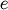)
NPY_LOG2E
Logarithm to base 2 of the Euler constant (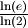)
NPY_LOG10E
Logarithm to base 10 of the Euler constant (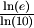)
NPY_LOGE2
Natural logarithm of 2 (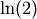)
NPY_LOGE10
Natural logarithm of 10 (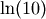)
NPY_PI
Pi (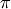)
NPY_PI_2
Pi divided by 2 (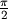)
NPY_PI_4
Pi divided by 4 (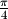)
NPY_1_PI
Reciprocal of pi (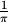)
NPY_2_PI
Two times the reciprocal of pi (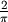)

UFunc API

Numpy internals